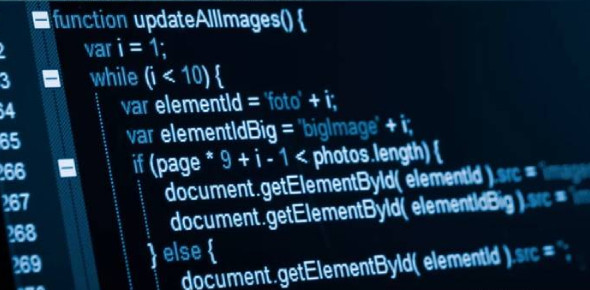# C Programming Exam Quiz!

40 Questions | Attempts: 687
ShareSettingsDo you understand C Programming? It is often a wellspring of confusion for those who are not as advanced with computers. The focus here is identifying a loop construct, storage class, what is in a switch statement, and when the global variable can be declared. You must know a fair amount of mathematics to understand C Programming. If you are a computer major, you will not be disappointed with this awesome quiz.

• 1.
Identify the loop construct:
• A.

If-else

• B.

Goto

• C.

While

• D.

Switch-case

• 2.
Which of the following is not a storage class?
• A.

External

• B.

Automatic

• C.

Register

• D.

Define

• 3.
Which of the following is a correct way of defining a symbolic constant pie in C?
• A.

# define pie = 22/7

• B.

#define pie 22/7

• C.

#define pie= 3.142

• D.

# Define pie 22/7

• 4.
The minimum number of times the for loop is executed is:
• A.

0

• B.

Cannot be predicted

• C.

1

• D.

2

• 5.
In switch statement:
• A.

More than one default allowesd

• B.

Default case, if used, should be the last case

• C.

Default case, if used, can be placed anywhere

• D.

Default case must be present

• 6.
How many while statements are possible in do... While loop?
• A.

2

• B.

3

• C.

Any number

• D.

1

• 7.
The storage area for register variables.
• A.

Cache

• B.

Memory

• C.

Processor registers

• D.

Virtual memory

• 8.
Which of the following statements is false?
• A.

The initialization and increment parts of a for statement can be empty

• B.

The body of do-while statement can be empty

• C.

The expression in the condition part of a for statement can be empty

• D.

The initialization part of a for statement cannot have more than one initialization

• 9.
Consider the segment If(1) printf(“yes”); else printf(“no”); what will be the output
• A.

No

• B.

Unpredictable

• C.

Yes

• D.

Error

• 10.
The global variable can be declared:
• A.

After main

• B.

After block

• C.

Before main

• D.

Within block

• 11.
Which of the following is not correct?
• A.

While loop is executed atleast once

• B.

Do . while loop is executed at least once

• C.

While loop is executed only if the condition is true

• D.

Dowhile loop is ececuted only if the condition is true

• 12.
Printf (“\ “ well done\” ”); what will be the output of this statement?
• A.

\“well done \”

• B.

Well done

• C.

“ well done”

• D.

\ well done \

• 13.
What is the output of the following program? main() {static int y; printf (“%d\n”, y); }
• A.

0

• B.

Run-time error

• C.

Undefined

• D.

Compilation error

• 14.
The number of elements in array declaration:
• A.

Dynamically identifies based on largest index used in program

• B.

Does not require to be specified

• C.

Assume default size as ‘0’

• D.

Requires to be specified

• 15.
In C every variable has:
• A.

A type and size

• B.

A type, name, value and size

• C.

A name and type

• D.

A size and value

• 16.
All the elements in the array must be:
• A.

Defined

• B.

Neither initialized nor defined

• C.

Initialized and defined

• D.

Initialized

• 17.
How many bytes will be allotted for the declaration int num ?
• A.

6 bytes

• B.

24 bytes

• C.

12 bytes

• D.

48 bytes

• 18.
If statement is a —————statement.
• A.

Loop construct

• B.

Two way decision

• C.

Multiway decision

• D.

One-way decision

• 19.
The total memory required for an array:
• A.

Sizeof (datatype) * 2

• B.

Size of (datatype) * size of used array elements

• C.

Sizeof (datatype) * sizeof array

• D.

Size of (array) * datatype

• 20.
Int table  ={{0}, {0}} in this statement.
• A.

None of the element are initialized to zero

• B.

Only first row elements are initialized to zero

• C.

Only last row elements are initialized to zero

• D.

All the array elements are initialized to zero

• 21.
The statement that prints out the character set from A-Z is, where a is an integer variable.
• A.

For(a=‘A’; a

• B.

For(a=‘A’; a

• C.

For(a=‘a’; a

• D.

For(a=’A’ a

• 22.
The amount of storage required for holding elements of the array depends on:
• A.

Data type

• B.

Datatype and size

• C.

Run-time requirement

• D.

Size

• 23.
If we don’t initialize a static array, what will be the elements set to:
• A.

Character constant

• B.

A floating point number

• C.

0

• D.

An undetermined value

• 24.
What is wrong with the following program? main() { char m1= “message1”; char m2=“message2”; m2=m1; printf(“msg is %s”,m2); }
• A.

Char array cannot be printed directly using printf

• B.

Array is not a left value and so cannot be assigned to

• C.

Program compiles without error, but prints an unpredictable value

• D.

Array cannot be initialized as above

• 25.
Array subscripts in ‘C’ always start at:
• A.

0

• B.

Compiler dependent

• C.

1

• D.

-1

## Related TopicsBack to top
×

Wait!
Here's an interesting quiz for you.